This book is archived and will be removed July 6, 2022. Please use the updated version.

Diffraction

# 29 Circular Apertures and Resolution

### Learning Objectives

By the end of this section, you will be able to:

• Describe the diffraction limit on resolution
• Describe the diffraction limit on beam propagation

Light diffracts as it moves through space, bending around obstacles, interfering constructively and destructively. This can be used as a spectroscopic tool—a diffraction grating disperses light according to wavelength, for example, and is used to produce spectra—but diffraction also limits the detail we can obtain in images.

(Figure)(a) shows the effect of passing light through a small circular aperture. Instead of a bright spot with sharp edges, we obtain a spot with a fuzzy edge surrounded by circles of light. This pattern is caused by diffraction, similar to that produced by a single slit. Light from different parts of the circular aperture interferes constructively and destructively. The effect is most noticeable when the aperture is small, but the effect is there for large apertures as well.

(a) Monochromatic light passed through a small circular aperture produces this diffraction pattern. (b) Two point-light sources that are close to one another produce overlapping images because of diffraction. (c) If the sources are closer together, they cannot be distinguished or resolved.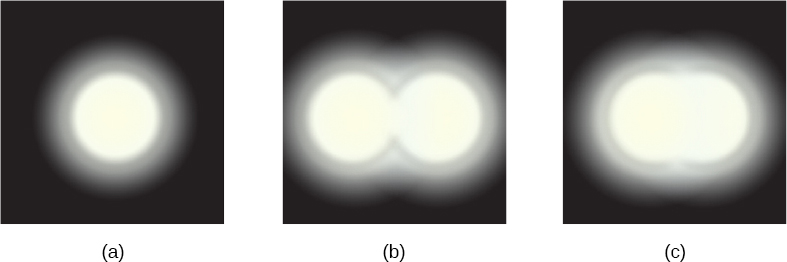How does diffraction affect the detail that can be observed when light passes through an aperture? (Figure)(b) shows the diffraction pattern produced by two point-light sources that are close to one another. The pattern is similar to that for a single point source, and it is still possible to tell that there are two light sources rather than one. If they are closer together, as in (Figure)(c), we cannot distinguish them, thus limiting the detail or resolution we can obtain. This limit is an inescapable consequence of the wave nature of light.

Diffraction limits the resolution in many situations. The acuity of our vision is limited because light passes through the pupil, which is the circular aperture of the eye. Be aware that the diffraction-like spreading of light is due to the limited diameter of a light beam, not the interaction with an aperture. Thus, light passing through a lens with a diameter D shows this effect and spreads, blurring the image, just as light passing through an aperture of diameter D does. Thus, diffraction limits the resolution of any system having a lens or mirror. Telescopes are also limited by diffraction, because of the finite diameter D of the primary mirror.

Just what is the limit? To answer that question, consider the diffraction pattern for a circular aperture, which has a central maximum that is wider and brighter than the maxima surrounding it (similar to a slit) ((Figure)(a)). It can be shown that, for a circular aperture of diameter D, the first minimum in the diffraction pattern occurs at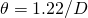(providing the aperture is large compared with the wavelength of light, which is the case for most optical instruments). The accepted criterion for determining the diffraction limit to resolution based on this angle is known as the Rayleigh criterion, which was developed by Lord Rayleigh in the nineteenth century.

Rayleigh Criterion

The diffraction limit to resolution states that two images are just resolvable when the center of the diffraction pattern of one is directly over the first minimum of the diffraction pattern of the other ((Figure)(b)).

The first minimum is at an angle of, so that two point objects are just resolvable if they are separated by the angle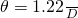whereis the wavelength of light (or other electromagnetic radiation) and D is the diameter of the aperture, lens, mirror, etc., with which the two objects are observed. In this expression,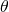has units of radians. This angle is also commonly known as the diffraction limit.

(a) Graph of intensity of the diffraction pattern for a circular aperture. Note that, similar to a single slit, the central maximum is wider and brighter than those to the sides. (b) Two point objects produce overlapping diffraction patterns. Shown here is the Rayleigh criterion for being just resolvable. The central maximum of one pattern lies on the first minimum of the other.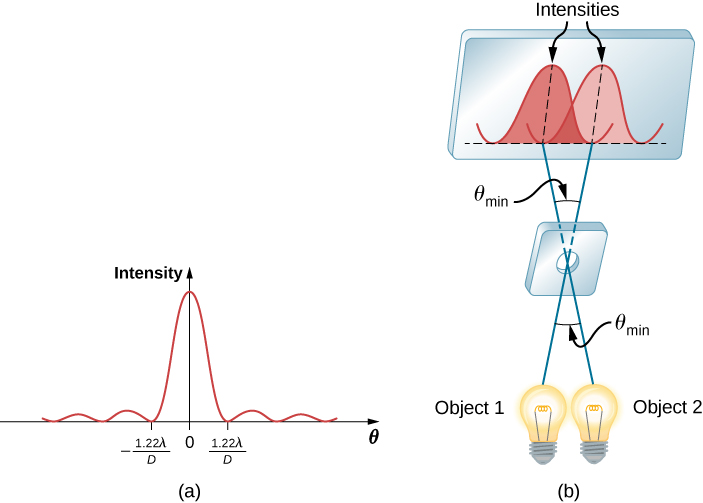All attempts to observe the size and shape of objects are limited by the wavelength of the probe. Even the small wavelength of light prohibits exact precision. When extremely small wavelength probes are used, as with an electron microscope, the system is disturbed, still limiting our knowledge. Heisenberg’s uncertainty principle asserts that this limit is fundamental and inescapable, as we shall see in the chapter on quantum mechanics.

Calculating Diffraction Limits of the Hubble Space Telescope The primary mirror of the orbiting Hubble Space Telescope has a diameter of 2.40 m. Being in orbit, this telescope avoids the degrading effects of atmospheric distortion on its resolution. (a) What is the angle between two just-resolvable point light sources (perhaps two stars)? Assume an average light wavelength of 550 nm. (b) If these two stars are at a distance of 2 million light-years, which is the distance of the Andromeda Galaxy, how close together can they be and still be resolved? (A light-year, or ly, is the distance light travels in 1 year.)

Strategy The Rayleigh criterion stated in (Figure),, gives the smallest possible anglebetween point sources, or the best obtainable resolution. Once this angle is known, we can calculate the distance between the stars, since we are given how far away they are.

Solution

1. The Rayleigh criterion for the minimum resolvable angle is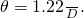Entering known values gives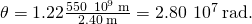2. The distance s between two objects a distance r away and separated by an angleis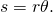Substituting known values gives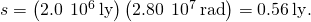Significance The angle found in part (a) is extraordinarily small (less than 1/50,000 of a degree), because the primary mirror is so large compared with the wavelength of light. As noticed, diffraction effects are most noticeable when light interacts with objects having sizes on the order of the wavelength of light. However, the effect is still there, and there is a diffraction limit to what is observable. The actual resolution of the Hubble Telescope is not quite as good as that found here. As with all instruments, there are other effects, such as nonuniformities in mirrors or aberrations in lenses that further limit resolution. However, (Figure) gives an indication of the extent of the detail observable with the Hubble because of its size and quality, and especially because it is above Earth’s atmosphere.

These two photographs of the M82 Galaxy give an idea of the observable detail using (a) a ground-based telescope and (b) the Hubble Space Telescope. (credit a: modification of work by “Ricnun”/Wikimedia Commons; credit b: modification of work by NASA, ESA, and The Hubble Heritage Team (STScI/AURA))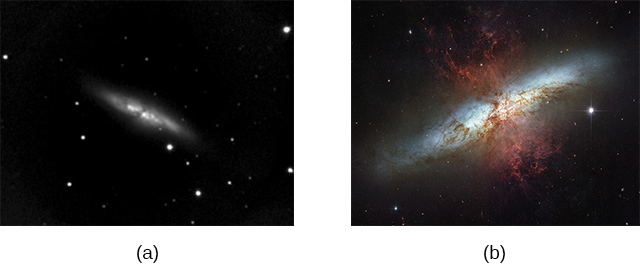The answer in part (b) indicates that two stars separated by about half a light-year can be resolved. The average distance between stars in a galaxy is on the order of five light-years in the outer parts and about one light-year near the galactic center. Therefore, the Hubble can resolve most of the individual stars in Andromeda Galaxy, even though it lies at such a huge distance that its light takes 2 million years to reach us. (Figure) shows another mirror used to observe radio waves from outer space.

A 305-m-diameter paraboloid at Arecibo in Puerto Rico is lined with reflective material, making it into a radio telescope. It is the largest curved focusing dish in the world. Although D for Arecibo is much larger than for the Hubble Telescope, it detects radiation of a much longer wavelength and its diffraction limit is significantly poorer than Hubble’s. The Arecibo telescope is still very useful, because important information is carried by radio waves that is not carried by visible light. (credit: Jeff Hitchcock)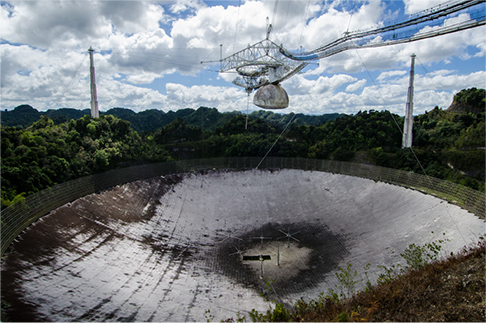Check Your Understanding What is the angular resolution of the Arecibo telescope shown in (Figure) when operated at 21-cm wavelength? How does it compare to the resolution of the Hubble Telescope?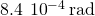, 3000 times broader than the Hubble Telescope

Diffraction is not only a problem for optical instruments but also for the electromagnetic radiation itself. Any beam of light having a finite diameter D and a wavelengthexhibits diffraction spreading. The beam spreads out with an anglegiven by (Figure),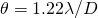. Take, for example, a laser beam made of rays as parallel as possible (angles between rays as close to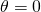as possible) instead spreads out at an angle, where D is the diameter of the beam andis its wavelength. This spreading is impossible to observe for a flashlight because its beam is not very parallel to start with. However, for long-distance transmission of laser beams or microwave signals, diffraction spreading can be significant ((Figure)). To avoid this, we can increase D. This is done for laser light sent to the moon to measure its distance from Earth. The laser beam is expanded through a telescope to make D much larger andsmaller.

The beam produced by this microwave transmission antenna spreads out at a minimum angledue to diffraction. It is impossible to produce a near-parallel beam because the beam has a limited diameter.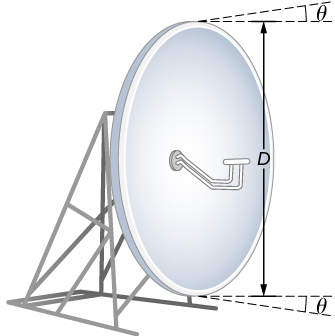In most biology laboratories, resolution is an issue when the use of the microscope is introduced. The smaller the distance x by which two objects can be separated and still be seen as distinct, the greater the resolution. The resolving power of a lens is defined as that distance x. An expression for resolving power is obtained from the Rayleigh criterion. (Figure)(a) shows two point objects separated by a distance x. According to the Rayleigh criterion, resolution is possible when the minimum angular separation is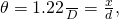where d is the distance between the specimen and the objective lens, and we have used the small angle approximation (i.e., we have assumed that x is much smaller than d), so that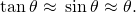Therefore, the resolving power is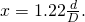Another way to look at this is by the concept of numerical aperture (NA), which is a measure of the maximum acceptance angle at which a lens will take light and still contain it within the lens. (Figure)(b) shows a lens and an object at point P. The NA here is a measure of the ability of the lens to gather light and resolve fine detail. The angle subtended by the lens at its focus is defined to be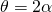. From the figure and again using the small angle approximation, we can write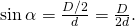The NA for a lens is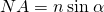, where n is the index of refraction of the medium between the objective lens and the object at point P. From this definition for NA, we can see that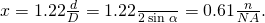In a microscope, NA is important because it relates to the resolving power of a lens. A lens with a large NA is able to resolve finer details. Lenses with larger NA are also able to collect more light and so give a brighter image. Another way to describe this situation is that the larger the NA, the larger the cone of light that can be brought into the lens, so more of the diffraction modes are collected. Thus the microscope has more information to form a clear image, and its resolving power is higher.

(a) Two points separated by a distance x and positioned a distance d away from the objective. (b) Terms and symbols used in discussion of resolving power for a lens and an object at point P (credit a: modification of work by “Infopro”/Wikimedia Commons).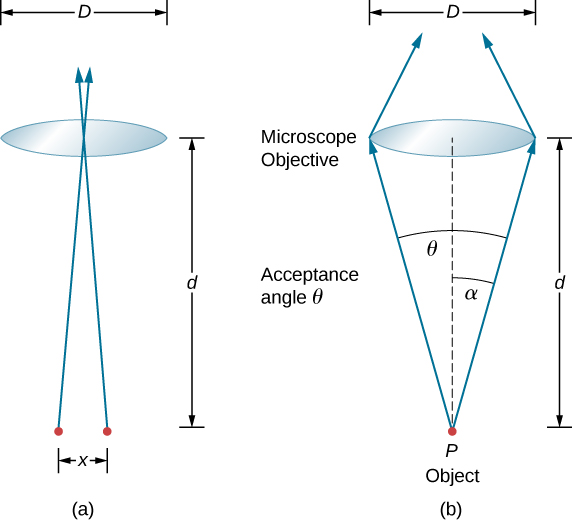One of the consequences of diffraction is that the focal point of a beam has a finite width and intensity distribution. Imagine focusing when only considering geometric optics, as in (Figure)(a). The focal point is regarded as an infinitely small point with a huge intensity and the capacity to incinerate most samples, irrespective of the NA of the objective lens—an unphysical oversimplification. For wave optics, due to diffraction, we take into account the phenomenon in which the focal point spreads to become a focal spot ((Figure)(b)) with the size of the spot decreasing with increasing NA. Consequently, the intensity in the focal spot increases with increasing NA. The higher the NA, the greater the chances of photodegrading the specimen. However, the spot never becomes a true point.

(a) In geometric optics, the focus is modelled as a point, but it is not physically possible to produce such a point because it implies infinite intensity. (b) In wave optics, the focus is an extended region.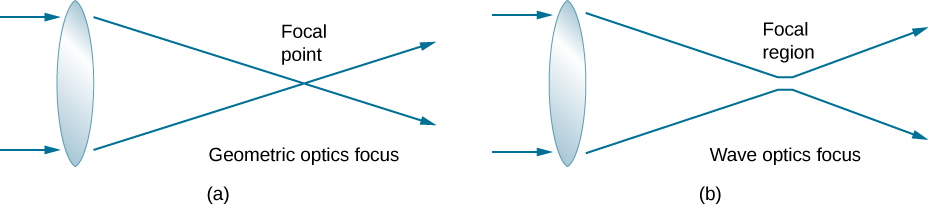In a different type of microscope, molecules within a specimen are made to emit light through a mechanism called fluorescence. By controlling the molecules emitting light, it has become possible to construct images with resolution much finer than the Rayleigh criterion, thus circumventing the diffraction limit. The development of super-resolved fluorescence microscopy led to the 2014 Nobel Prize in Chemistry.

In this Optical Resolution Model, two diffraction patterns for light through two circular apertures are shown side by side in this simulation by Fu-Kwun Hwang. Watch the patterns merge as you decrease the aperture diameters.

### Summary

• Diffraction limits resolution.
• The Rayleigh criterion states that two images are just resolvable when the center of the diffraction pattern of one is directly over the first minimum of the diffraction pattern of the other.

### Conceptual Questions

Is higher resolution obtained in a microscope with red or blue light? Explain your answer.

blue; The shorter wavelength of blue light results in a smaller angle for diffraction limit.

The resolving power of refracting telescope increases with the size of its objective lens. What other advantage is gained with a larger lens?

The distance between atoms in a molecule is about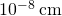. Can visible light be used to “see” molecules?

No, these distances are three orders of magnitude smaller than the wavelength of visible light, so visible light makes a poor probe for atoms.

A beam of light always spreads out. Why can a beam not be created with parallel rays to prevent spreading? Why can lenses, mirrors, or apertures not be used to correct the spreading?

### Problems

The 305-m-diameter Arecibo radio telescope pictured in (Figure) detects radio waves with a 4.00-cm average wavelength. (a) What is the angle between two just-resolvable point sources for this telescope? (b) How close together could these point sources be at the 2 million light-year distance of the Andromeda Galaxy?

Assuming the angular resolution found for the Hubble Telescope in (Figure), what is the smallest detail that could be observed on the moon?

107 m

Diffraction spreading for a flashlight is insignificant compared with other limitations in its optics, such as spherical aberrations in its mirror. To show this, calculate the minimum angular spreading of a flashlight beam that is originally 5.00 cm in diameter with an average wavelength of 600 nm.

(a) What is the minimum angular spread of a 633-nm wavelength He-Ne laser beam that is originally 1.00 mm in diameter? (b) If this laser is aimed at a mountain cliff 15.0 km away, how big will the illuminated spot be? (c) How big a spot would be illuminated on the moon, neglecting atmospheric effects? (This might be done to hit a corner reflector to measure the round-trip time and, hence, distance.)

a.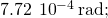b. 23.2 m; c. 590 km

A telescope can be used to enlarge the diameter of a laser beam and limit diffraction spreading. The laser beam is sent through the telescope in opposite the normal direction and can then be projected onto a satellite or the moon. (a) If this is done with the Mount Wilson telescope, producing a 2.54-m-diameter beam of 633-nm light, what is the minimum angular spread of the beam? (b) Neglecting atmospheric effects, what is the size of the spot this beam would make on the moon, assuming a lunar distance of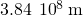?

The limit to the eye’s acuity is actually related to diffraction by the pupil. (a) What is the angle between two just-resolvable points of light for a 3.00-mm-diameter pupil, assuming an average wavelength of 550 nm? (b) Take your result to be the practical limit for the eye. What is the greatest possible distance a car can be from you if you can resolve its two headlights, given they are 1.30 m apart? (c) What is the distance between two just-resolvable points held at an arm’s length (0.800 m) from your eye? (d) How does your answer to (c) compare to details you normally observe in everyday circumstances?

a.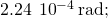b. 5.81 km; c. 0.179 mm; d. can resolve details 0.2 mm apart at arm’s length

What is the minimum diameter mirror on a telescope that would allow you to see details as small as 5.00 km on the moon some 384,000 km away? Assume an average wavelength of 550 nm for the light received.

Find the radius of a star’s image on the retina of an eye if its pupil is open to 0.65 cm and the distance from the pupil to the retina is 2.8 cm. Assume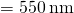.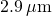(a) The dwarf planet Pluto and its moon, Charon, are separated by 19,600 km. Neglecting atmospheric effects, should the 5.08-m-diameter Palomar Mountain telescope be able to resolve these bodies when they are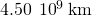from Earth? Assume an average wavelength of 550 nm. (b) In actuality, it is just barely possible to discern that Pluto and Charon are separate bodies using a ground-based telescope. What are the reasons for this?

A spy satellite orbits Earth at a height of 180 km. What is the minimum diameter of the objective lens in a telescope that must be used to resolve columns of troops marching 2.0 m apart? Assume6.0 cm

What is the minimum angular separation of two stars that are just-resolvable by the 8.1-m Gemini South telescope, if atmospheric effects do not limit resolution? Use 550 nm for the wavelength of the light from the stars.

The headlights of a car are 1.3 m apart. What is the maximum distance at which the eye can resolve these two headlights? Take the pupil diameter to be 0.40 cm.

7.71 km

When dots are placed on a page from a laser printer, they must be close enough so that you do not see the individual dots of ink. To do this, the separation of the dots must be less than Raleigh’s criterion. Take the pupil of the eye to be 3.0 mm and the distance from the paper to the eye of 35 cm; find the minimum separation of two dots such that they cannot be resolved. How many dots per inch (dpi) does this correspond to?

Suppose you are looking down at a highway from a jetliner flying at an altitude of 6.0 km. How far apart must two cars be if you are able to distinguish them? Assume thatand that the diameter of your pupils is 4.0 mm.

1.0 m

Can an astronaut orbiting Earth in a satellite at a distance of 180 km from the surface distinguish two skyscrapers that are 20 m apart? Assume that the pupils of the astronaut’s eyes have a diameter of 5.0 mm and that most of the light is centered around 500 nm.

The characters of a stadium scoreboard are formed with closely spaced lightbulbs that radiate primarily yellow light. (Use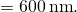) How closely must the bulbs be spaced so that an observer 80 m away sees a display of continuous lines rather than the individual bulbs? Assume that the pupil of the observer’s eye has a diameter of 5.0 mm.

1.2 cm or closer

If a microscope can accept light from objects at angles as large as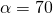, what is the smallest structure that can be resolved when illuminated with light of wavelength 500 nm and (a) the specimen is in air? (b) When the specimen is immersed in oil, with index of refraction of 1.52?

A camera uses a lens with aperture 2.0 cm. What is the angular resolution of a photograph taken at 700 nm wavelength? Can it resolve the millimeter markings of a ruler placed 35 m away?

no

### Glossary

diffraction limit
fundamental limit to resolution due to diffraction
Rayleigh criterion
two images are just-resolvable when the center of the diffraction pattern of one is directly over the first minimum of the diffraction pattern of the other
resolution
ability, or limit thereof, to distinguish small details in images